# About Congruent Triangles

In school, we all learned the conditions for determining the congruence of triangles. There are usually 5 kinds of things mentioned in the textbook : $SSS,SAS,AAS,ASA,HL$. We notice that the above conditions are all around angles and edges, but there are other lines and points, such as Angle bisectors, heights, centers, etc. Can we use other conditions to determine the congruence of triangles? I made three proofs by myself. But there are too many of these to do it myself. If anyone has a great idea, let me know in the comments section and I'll add it to my note.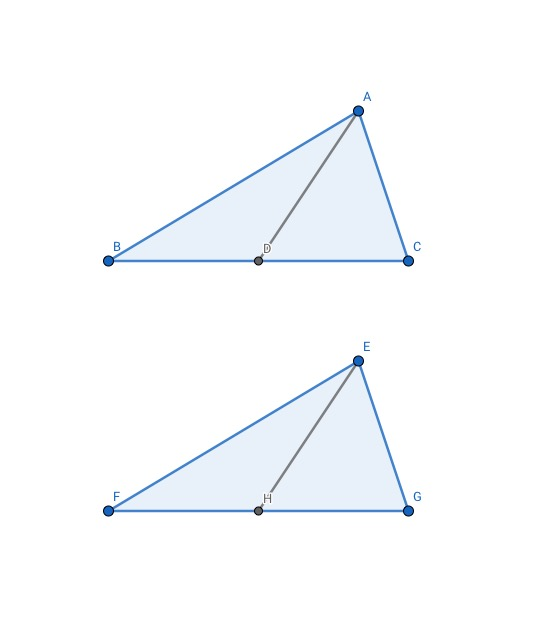When two triangle have two equal edges and one side has an equal midian,they are congruent figures.

In the picture, $AC=EG$,$BC=FG$, $AD$ and $EH$ are midians on $BC$ and $FG$.

$\because D$ and $H$ are midpoint on $BC$ and $FG$, there are $BC=FG$. $\implies CD=GH,BD=FH$.

In the $\triangle ACD$ and $\triangle EGH$, $AD=EH,AC=EG,GH=CD$

$\therefore \triangle ACD \cong \triangle EGH(SSS)\implies\angle ACB=\angle EGF$

In the $\triangle ABC$ and $\triangle EFG$, $AC=EG,\angle ACB=\angle EGF,BC=FG$

$\therefore \triangle ABC\cong \triangle EFG(SAS)$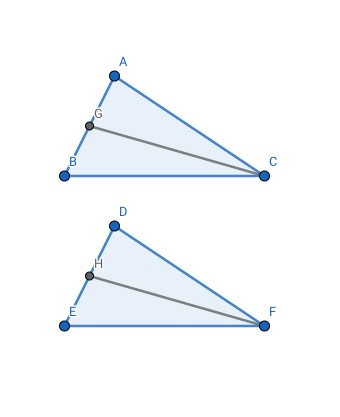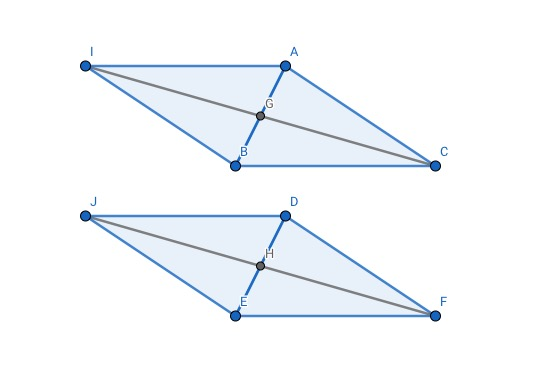When two triangles have two equal edges and the other side has an equal midian, they are congruent figures.

In the picture, $AC=DF$, $BC=EF$, $CG$ and $FH$ are midians on $AB$ and $DE$

We extend $CG$ and $FH$ to $I$ and $J$, and let $CG=IJ=\dfrac12 CI, FH=JH=\dfrac12 FJ$.

$\implies G$ and $H$ are midpoints on $CI$ and $FG$. Also, $G$ and $H$ are midpoint on $AB$ and $DE$.

$\implies AIBC$ and $DJEF$ are parallelograms. $\implies AC=BI, DF=EJ\implies BI=EJ$

$\because AC=DF, BC=EF, CG=FH \implies 2CG=2FH \implies CI=FJ$

In the $\triangle BCI$ and $\triangle EFJ$, $BC=EF, CI=FJ, BI=EJ$

$\therefore \triangle BCI \cong \triangle EFJ(SSS) \implies \angle EFJ=\angle BCI$

Also, we can know $\triangle ACI\cong \triangle DFJ(SSS) \implies \angle ACI=\angle DFJ$

$\therefore \angle ACI+\angle BCI=\angle DFJ+\angle EFJ\implies \angle ACB=\angle DFE$

In the $\triangle ABC$ and $\triangle DEF$,$AC=DF, \angle ACB=\angle DFE, BC=EF$

$\therefore \triangle ABC\cong \triangle DEF(SAS)$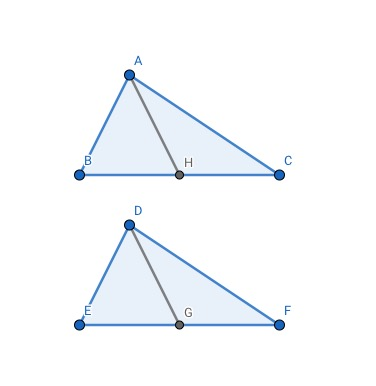When two triangles have two equal angles and they clip edge has an equal midian, they're congruent figures.

In the picture, $\angle B=\angle E, \angle C=\angle F,AH$ and $DG$ are midians on $BC$ and $EF$

In the $\triangle ABC$ and $\triangle DEF$, $\angle B=\angle E, \angle C=\angle F$

$\therefore \triangle ABC \sim \triangle DEF (AA) \implies \dfrac{AC}{DF}=\dfrac{AB}{DE}=\dfrac{BC}{EF}$

$\because AH=DG \implies \dfrac{AH}{DG}=\dfrac{BC}{EF}=1 \implies BC=EF$

In the $\triangle ABC$ and $\triangle DEF$, $\angle B=\angle E, \angle C=\angle F, BC=EF$

$\therefore \triangle ABC\cong \triangle DEF(ASA)$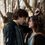Note by Edward Christian
1 year, 9 months ago

This discussion board is a place to discuss our Daily Challenges and the math and science related to those challenges. Explanations are more than just a solution — they should explain the steps and thinking strategies that you used to obtain the solution. Comments should further the discussion of math and science.

When posting on Brilliant:

• Use the emojis to react to an explanation, whether you're congratulating a job well done , or just really confused .
• Ask specific questions about the challenge or the steps in somebody's explanation. Well-posed questions can add a lot to the discussion, but posting "I don't understand!" doesn't help anyone.
• Try to contribute something new to the discussion, whether it is an extension, generalization or other idea related to the challenge.
• Stay on topic — we're all here to learn more about math and science, not to hear about your favorite get-rich-quick scheme or current world events.

MarkdownAppears as
*italics* or _italics_ italics
**bold** or __bold__ bold
- bulleted- list
• bulleted
• list
1. numbered2. list
1. numbered
2. list
Note: you must add a full line of space before and after lists for them to show up correctly
paragraph 1paragraph 2

paragraph 1

paragraph 2

[example link](https://brilliant.org)example link
> This is a quote
This is a quote
    # I indented these lines
# 4 spaces, and now they show
# up as a code block.

print "hello world"
# I indented these lines
# 4 spaces, and now they show
# up as a code block.

print "hello world"
MathAppears as
Remember to wrap math in $$ ... $$ or $ ... $ to ensure proper formatting.
2 \times 3 $2 \times 3$
2^{34} $2^{34}$
a_{i-1} $a_{i-1}$
\frac{2}{3} $\frac{2}{3}$
\sqrt{2} $\sqrt{2}$
\sum_{i=1}^3 $\sum_{i=1}^3$
\sin \theta $\sin \theta$
\boxed{123} $\boxed{123}$

## Comments

Sort by:

Top Newest

Creative Design ........... Thanks, mate for sharing this. I left mathematics a long time ago although it was interesting for me. I loved to know these facts.

- 1 year, 2 months ago

Log in to reply

×

Problem Loading...

Note Loading...

Set Loading...